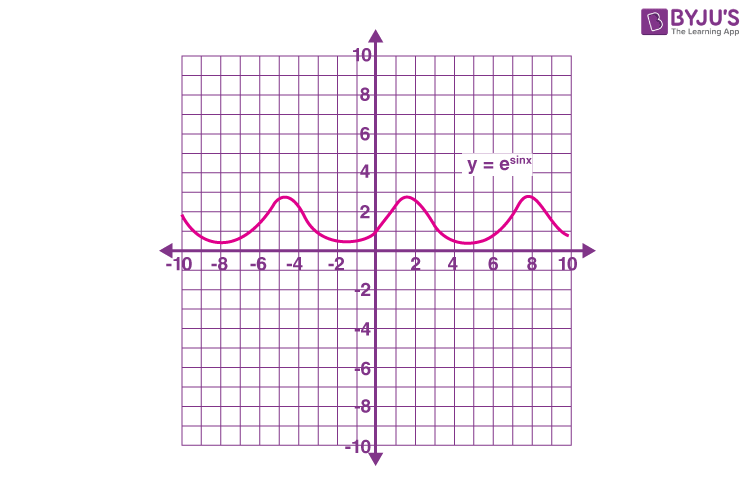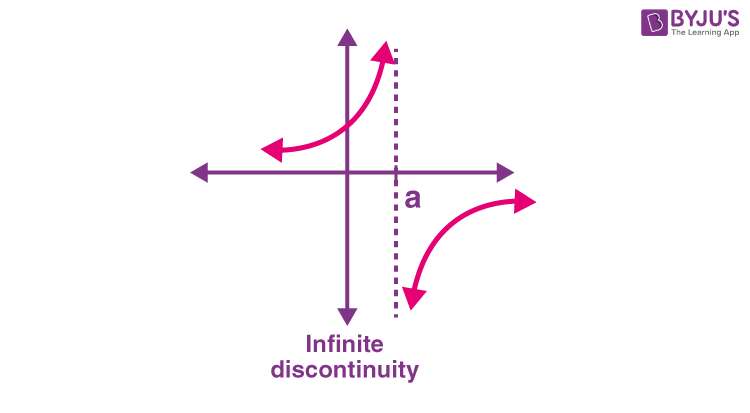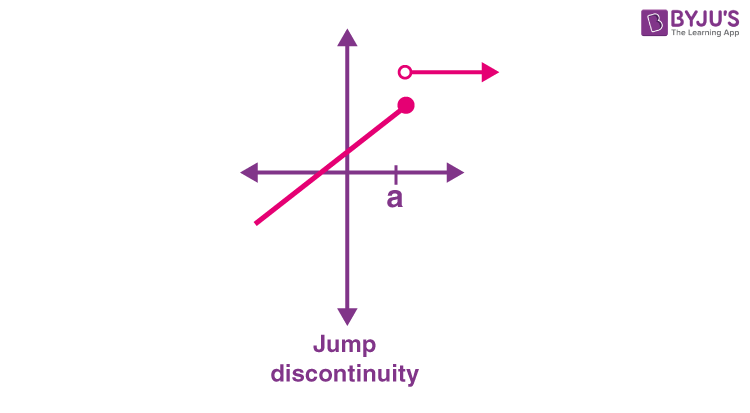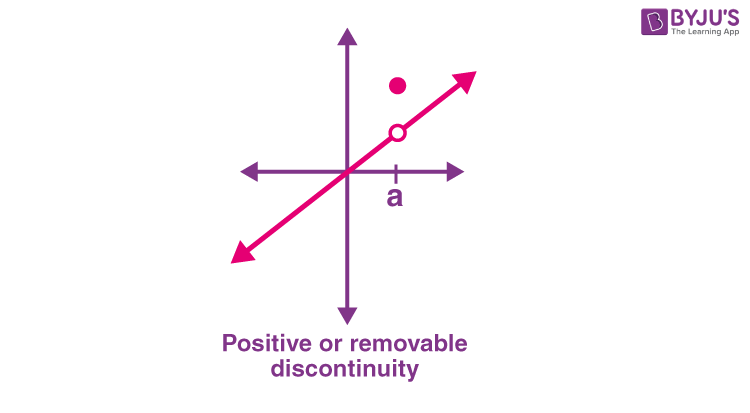# Limits And Continuity

Limits and continuity concept is one of the most crucial topics in calculus. Combinations of these concepts have been widely explained in Class 11 and Class 12. A limit is defined as a number approached by the function as an independent function’s variable approaches a particular value. For instance, for a function f(x) = 4x, you can say that “The limit of f(x) as x approaches 2 is 8”. Symbolically, it is written as;

$$\begin{array}{l}\lim \limits_{x \to 2} (4x) = 4 \times 2 = 8\end{array}$$

Continuity is another popular topic in calculus. The easy method to test for the continuity of a function is to examine whether a pen can trace the graph of a function without lifting the pen from the paper. When you are doing precalculus and calculus, a conceptual definition is almost sufficient, but for higher level, a technical explanation is required. You can learn a better and precise way of defining continuity by using limits.

## Continuity Definition

Many functions have the property that they can trace their graphs with a pencil without lifting the pencil from the paper’s surface. These types of functions are called continuous. Intuitively, a function is continuous at a particular point if there is no break in its graph at that point. A precise definition of continuity of a real function is provided generally in a calculus’s introductory course in terms of a limit’s idea. First, a function f with variable x is continuous at the point “a” on the real line, if the limit of f(x), when x approaches the point “a”, is equal to the value of f(x) at “a”, i.e., f(a). Second, the function (as a whole) is continuous, if it is continuous at every point in its domain.

Mathematically, continuity can be defined as given below:

A function is said to be continuous at a particular point if the following three conditions are satisfied.

1. f(a) is defined
2. $$\begin{array}{l}\lim\limits_{x \to a} f(x) \text{ exists}\end{array}$$
3. $$\begin{array}{l}\lim\limits_{x \to a^{+}}f(x)= \lim\limits_{x \to a^{-}}f(x)= f(a)\end{array}$$

As mentioned before, a function is said to be continuous if you can trace its graph without lifting the pen from the paper. But a function is said to be discontinuous when it has any gap in between.

Below figure shows the graph of a continuous function.## Types of Discontinuity

There are basically two types of discontinuity:

• Infinite Discontinuity
• Jump Discontinuity

### Infinite Discontinuity

A branch of discontinuity wherein, a vertical asymptote is present at x = a and f(a) is not defined. This is also called Asymptotic Discontinuity. If a function has values on both sides of an asymptote, then it cannot be connected, so it is discontinuous at the asymptote. This can be shown using the graph as given below.### Jump Discontinuity

$$\begin{array}{l}\text{A branch of discontinuity wherein } \lim\limits_{x \to a^{+}}f(x) \neq \lim\limits_{x \to a^{-}}f(x)\text{, but both the limits are finite.}\end{array}$$

This is also called simple discontinuity or continuities of the first kind. The graphical representation of jump discontinuity is given below.### Positive Discontinuity

A branch of discontinuity wherein a function has a predefined two-sided limit at x = a, but either f(x) is undefined at a, or its value is not equal to the limit at a. This is also called a removable discontinuity.

Graphically, this can be shown as:## Limit Definition

A limit of a function is a number that a function reaches as the independent variable of the function reaches a given value. The value (say a) to which the function f(x) gets close arbitrarily as the value of the independent variable x becomes close arbitrarily to a given value “A” symbolized as f(x) = A.

Points to remember:

• If limx→a- f(x) is the expected value of f at x = a given the values of ‘f’ near x to the left of a. This value is known as the left-hand limit of ‘f’ at a.
• If limx→a+ f(x) is the expected value of f at x = a given the values of ‘f’ near x to the right of a. This value is known as the right-hand limit of f(x) at a.
• If the right-hand and left-hand limits coincide, we say the common value as the limit of f(x) at x = a and denote it by limx→a f(x).

### One-Sided Limit

The limit that is based completely on the values of a function taken at x -value that is slightly greater or less than a particular value.

$$\begin{array}{l}\text{A two-sided limit } \lim\limits_{x \to a}f(x) \text{ takes the values of x into account that are both larger than and} \\ \text{ smaller than a.}\end{array}$$
$$\begin{array}{l}\text{A one-sided limit from the left }\lim\limits_{x \to a^{-}}f(x) \text{ or from the right} \lim\limits_{x \to a^{-}}f(x) \text{ takes only values of x} \\ \text{ smaller or greater than a respectively.}\end{array}$$

### Properties of Limit

• The limit of a function is represented as f(x) reaches L as x tends to limit a, such that; limx→af(x) = L
• The limit of the sum of two functions is equal to the sum of their limits, such that: limx→a [f(x) + g(x)] =  limx→a f(x) + limx→a g(x)
• The limit of any constant function is a constant term, such that, limx→a C = C
• The limit of product of the constant and function is equal to the product of constant and the limit of the function, such that: limx→a m f(x) = m limx→a f(x)
• Quotient Rule: limx→a[f(x)/g(x)] =  limx→af(x)/limx→ag(x); if limx→ag(x) ≠ 0

## Recommended Video

### Limits Class 11 Maths in One-Shot### Examples

$$\begin{array}{l}\text{1). Compute }\lim\limits_{x \to -2} \left ( 3x^{2}+5x-9 \right )\end{array}$$

Solution:

First, use property 2 to divide the limit into three separate limits. Then use property 1 to bring the constants out of the first two. This gives,

$$\begin{array}{l}\lim\limits_{x \to -2} \left ( 3x^{2}+5x-9 \right ) = \lim\limits_{x \to -2}(3x^{2}) + \lim\limits_{x \to -2}(5x) -\lim\limits_{x \to -2}(9)\end{array}$$

$$\begin{array}{l}= 3(-2)^{2} + 5(-2) -(9)\end{array}$$

= 12 – 10 – 9

= -7

2). Find the value of limx→3 [x(x+2)].

Solution:

limx→3 [x(x+2)] = 3(3+2) = 3 x 5 = 15

To know more about Limits and Continuity, Calculus, Differentiation etc. and solved examples, visit our site BYJU’S.

## Frequently Asked Questions on Limits and Continuity – FAQs

Q1

### How do you find the limit and continuity of a function?

We know that the value of f near x to the left of a, i.e. left-hand limit of f at a and the value of f near x to the right f a, i.e. right-hand limit are equal, then that common value is called the limit of f(x) at x = a. Also, a function f is said to be continuous at a if limit of f(x) as x approaches a is equal to f(a).
Q2

### How are limits related to continuity?

The definition of continuity is given with the help of limits as, a function f with variable x is continuous at the point “a” on the real line, if the limit of f(x), when x approaches the point “a”, is equal to the value of f(x) at “a”, that means f(a).
Q3

### What are the 3 conditions of continuity?

A function is said to be continuous at a particular point if the following three conditions are satisfied.
1. f(a) is defined
2. lim_{x→a} f(x) exists
3. lim_{x→a+} f(x) = lim_{x→a-} f(x) = f(a)
Q4

### What is limit and continuity in calculus?

Limits are used to make all the basic definitions of calculus. For example, limits are used to define continuous functions. The conventional definition of a limit implies that every function is continuous at every solitary point of its domain.
Q5

### What is the concept of continuity?

In general, continuity means the fact of not stopping or not changing. Continuity refers to something occurring in an uninterrupted state or on a regular and ongoing basis.